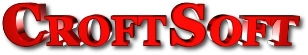CroftSoft / About / Contract / Library // Books // Code // Courses // Links // Media // Tutorials / People // David /// Resume // Shannon / Portfolio / Update## Why Exponential E?

David Wallace Croft

2004-10-22

## Purpose

The purpose of this webpage is to document why the exponential e (ⅇ) shows up in the solution to linear differential equations. This question has bothered me since I was in college back in the late 1980's and I only just recently got around to figuring out why. I suspect I may have figured this out at least once over the intervening years but then forgot the solution. By putting this on my website where I can find it, I hope to avoid duplication of my effort in the future. This proof is based on my own calculations and has not yet been confirmed with another source.

I had postponed putting my math notes on the web in the past because I wanted to wait until MathML was supported by one of the major Internet browsers. This finally happened with the release of Netscape 7.1 not too long ago.

This webpage serves as my first learning experience using MathML. You will need Mozilla or Netscape 7.1+ to view the MathML properly. Select View/Page Source (Control-U) to see the MathML code embedded in the XHTML. Select View/Text Zoom/Larger (Control-+) to increase the font size.

## Identities

1. $x\left(t+\Delta t\right)=\underset{k=0}{\overset{\infty }{\Sigma }}\frac{{\Delta t}^{k}}{k!}{x}^{\left(k\right)}\left(t\right)$ [Taylor Series]
2. ${e}^{\Delta t}=\underset{k=0}{\overset{\infty }{\Sigma }}\frac{{\Delta t}^{k}}{k!}$ [Exponential Function]

## Given

1. ${x}^{\text{'}}\left(t\right)=x\left(t\right)$ [Exponential Growth]

## Problem

1. $x\left(t+\Delta t\right)=?$

## Solution

1. ${x}^{\text{'}}\left(t\right)=x\left(t\right)$ [Exponential Growth]
2. ${x}^{\left(k\right)}\left(t\right)={x}^{\left(k-1\right)}\left(t\right)$ [for k > 0]
3. ${x}^{\left(k\right)}\left(t\right)=x\left(t\right)$ [for k > 0]
4. $x\left(t+\Delta t\right)=\underset{k=0}{\overset{\infty }{\Sigma }}\frac{{\Delta t}^{k}}{k!}{x}^{\left(k\right)}\left(t\right)$ [Taylor Series]
5. $x\left(t+\Delta t\right)=\underset{k=0}{\overset{\infty }{\Sigma }}\frac{{\Delta t}^{k}}{k!}x\left(t\right)$
6. $x\left(t+\Delta t\right)=x\left(t\right)\underset{k=0}{\overset{\infty }{\Sigma }}\frac{{\Delta t}^{k}}{k!}$
7. $x\left(t+\Delta t\right)=x\left(t\right){e}^{\Delta t}$